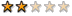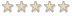## PP1+PP2 數學完整詳解版-2009.08.19更新 (更新者: sunnyblue13s)

### PP1+PP2 數學完整詳解版-2009.08.19更新 (更新者: sunnyblue13s)

DS1 - V1.1 / DS2 - V1.0
PS1 - V1.0 / PS2 - V1.0

sunnyblue13s筆

PP1+PP2作者群:
pauline_chiou, william0625, gman, yuma168 ,larry.tw, Huang Hsin-Yi, Penda, Engedi, mauricew, charlie6989, abc123, sunnyblue13s, ruru.lu

larry.tw### Re: PP1+PP2 數學完整詳解版 -- 勘誤表

[Philosophia ]

PP2-PS-Q176
x=4, y=-6, S is positive, 應是在第四象限，而非第二象限

PP2-DS-Q68

larry.tw### Re: PP1+PP2 數學完整詳解版 -- 勘誤表

PP2-DS-135

we need to find the value of P-T

St 1 tells us P+Q+R+S = 388250*4
P = 388250*4-Q-R-S
But since it doesnt tell us anything about T its INSUFF

St 2 tells us Q+R+S+T = 383000*4
T=383000*4-Q-R-S
but since it doesnt tell us anything about P its INSUFF

Combining them we get
P = 388250*4-Q-R-S
T=383000*4-Q-R-S

Subtract the two statements we get P-T = (388250*4)-(383000*4)
larry.tw### Re: PP1+PP2 數學完整詳解版 -- 勘誤表

PP2-DS-155

"500 is the multiple of 100 that's closest to x": this means that, of all multiples of 100, 500 is closest to x.
in other words, x is closer to 500 than to 400 or 600 (or to any other multiple of 100, for that matter). what does this mean?
it means that 450 < x < 550. (note that this is almost the same thing as "x rounded to the nearest hundred produces 500", except for the piddling exception of 450.)

(另一種說法:For 500 to be the multiple of 100 closest to x, x has to be between 450 and 550. If x is less than 450 e.g 438 then the multiple of 100 closest to x will be 400 and not 500. Similarly if x is greater than 550 e.g. 578 then the multiple of 100 closest to x will be 600 and not 500. )

the second half of the problem statement, similarly interpreted, gives the following: 350 < y < 450.

(1)
in combination with the question stem, this means that 450 < x < 500 (and we still know that 350 < y < 450).
therefore, 800 < x + y < 950.
this isn't good enough to answer the question; the numbers between 800 and 850 are closer to 800, the numbers between 850 and 950 are closer to 900, and 850 is equidistant from 800 and 900.
insufficient.

(2)
in combination with the question stem, this means that 350 < y < 400 (and we still know that 450 < x < 550).
therefore, 800 < x + y < 950, exactly the same inequality that holds for statement (1).
insufficient.

(together)
now we know that 450 < x < 500 and 350 < y < 400.
this means that 800 < x + y < 900.
still not good enough to answer the question; the numbers between 800 and 850 are closer to 800, the numbers between 850 and 900 are closer to 900, and 850 is equidistant from 800 and 900.
insufficient.

ans = e
larry.tw### Re: PP1+PP2 數學完整詳解版 -- 勘誤表

PP2-DS-184

(1)的條件 k/(2^6)=整數(設X)

(2)的條件 K不能被大於一的任何奇數整除

larry.tw### Re: PP1+PP2 數學完整詳解版 -- 勘誤表

PP2-DS-192

(1) + (2) => y - 2 < x < 2y - 6
y - 2 < 2y - 6, y > 4 => x > 2
=> xy > 0
=> (C)
larry.tw### Re: PP1+PP2 數學完整詳解版 -- 勘誤表

[文件]: PP1-PS
[題號]:
[內容]:補上Q5,6,11,13,14,15,17,18,25,28,29,31,37,38,46,47,50,55,56,59,61,62,63,67,72,78,80,83,87,91,92,93,97,
102,103,107,112,113,114,119,120,121,125,128,129,131,133,150,158,162,168,
173,176,177,179,182,187,191,190,193,等共60題，尚餘24題未齊，請大家繼續加油

http://www.4shared.com/file/71669175/e7 ... 1-V20.html

http://forum.chasedream.com/dispbbs.asp ... n=0&Star=1

http://www.4shared.com/file/70351665/8f4a3e6c/ds1.html
http://www.4shared.com/file/70352297/f36044bd/ds2.html
iamsmall### Re: PP1+PP2 數學完整詳解版-2009.08.19更新 (更新者: sunnyblue13s)

leafer### 誰在線上